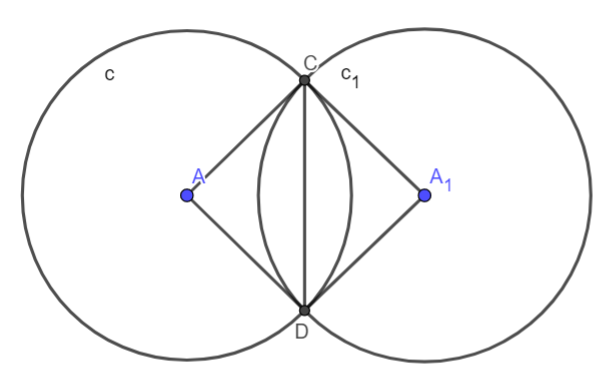﻿ 北京理工大学第七届程序设计新生赛 题解（unofficial） - 我的经验分享

xzm201912
2019/02

# 北京理工大学第七届程序设计新生赛 题解（unofficial）

SolvedABCDEFGHIJKLM
8/13ØO.OØOOØØOOOO
• O for passing during the contest
• Ø for passing after the contest
• ! for attempted but failed
• · for having not attempted yet

## Problem A 自然语言处理 (Unsolved with 13 tries)

$T(T \leq 100)$组数据，每组数据给你$n(1 \leq n \leq 10)$个$m(1 \leq m \leq 4)$维向量，请判断他们是否线性相关。

#include <bits/stdc++.h>
using namespace std;

const int maxn=505;
const double eps=1e-8;
double A[maxn<<1][maxn],x[maxn];
int n,m;
int Guass(int n,int m)
{
int i=1,j=1,k,r,c;
while(i<=m && j<=n)
{
r=i;
for(k=i+1;k<=m;k++)if(fabs(A[k][j])>fabs(A[r][j]))r=k;
if(fabs(A[r][j])>=eps)
{
for(c=1;c<=n+1;c++)swap(A[i][c],A[r][c]);
for(k=i+1;k<=m;k++)if(fabs(A[k][j])>=eps)
{
double f=A[k][j]/A[i][j];
for(c=j;c<=n+1;c++)
A[k][c]-=f*A[i][c];
}
i++;
}
j++;
}
for(k=i;k<=m;k++)if(fabs(A[k][n+1])>=eps)return 0;
if(i<=n)return 2;
for(int i=n;i>=1;i--)
{
for(j=i+1;j<=n;j++)
A[i][n+1]-=A[i][j]*x[j];
x[i]=A[i][n+1]/A[i][i];
}
return 1;
}
int main()
{
int T;
scanf("%d",&T);
while (T--)
{
scanf("%d%d",&n,&m);
memset(A,0,sizeof(A));
for (int i=1;i<=n;i++)
{
for (int j=1;j<=m;j++)
scanf("%lf",&A[j][i]);
}
if (n>m)
{
printf("YES\n"); continue;
}
m++;
A[m]=1; A[m][n+1]=1;
if (Guass(n,m)>=1) printf("YES\n");
else printf("NO\n");
}
return 0;
}

## Problem D 元素周期表 (Solved at 2:13 with 1 try)

$T(1 \leq T \leq 200)$组数据，每组数据给你一个长度小于1000分子式（分子式只包含元素和元素个数，没有括号、前导数字、点等），请求出它的相对分子质量。题目给出了一张元素周期表的图片。

## Problem E 龙语魔法 (Unattempted)

#include <bits/stdc++.h>
using namespace std;

int a;
int n;
long long k,all;
int check(long long x)
{
long long tmp1=0,tmp2=0,now=a;
int l=0,r=1;
while (l<=r&&r<=n)
{
if (now>=x)
{
if (now==x) tmp2++; else tmp1++;
tmp1+=n-r;
l++; now-=a[l];
}
else {r++; now+=a[r];}
}
if (all-tmp1-tmp2<k&&all-tmp1>=k) return 0;
if (all-tmp1-tmp2>=k) return 1;
else return -1;
}
int main()
{
scanf("%d%lld",&n,&k);
long long sum=0;
all=1LL*n*(n+1)/2;
for (int i=1;i<=n;i++) scanf("%d",&a[i]);
for (int i=1;i<=n;i++) sum+=a[i];
long long l=1,r=sum;
while (l<=r)
{
long long mid=(l+r)/2;
int flag=check(mid);
if (flag==0) return printf("%lld\n",mid),0;
if (flag==1) r=mid-1;
else l=mid+1;
}
return 0;
}

## Problem G 伙伴系统 (Solved at 1:48 with 2 tries)

$k(k \leq 10^5)$次操作，每次操作获得一块内存或者请求划分内存。内存要划分成二的次幂的内存块。每次划分不能把小的拼接，只能从大的再划分。

## Problem H 白学串 (Unattempted)

#include <bits/stdc++.h>
using namespace std;

int a;
int main()
{
int T;
scanf("%d",&T);
while (T--)
{
int n,m;
scanf("%d%d",&n,&m);
for (int i=1;i<=n;i++) scanf("%d",&a[i]);
while (m--)
{
int l,r;
scanf("%d%d",&l,&r);
if (r-l+1>=40) {printf("yes\n"); continue;}
vector<int> tmp;
for (int i=l;i<=r;i++)
tmp.emplace_back(a[i]);
sort(tmp.begin(),tmp.end());
bool flag=false;
for (int i=0;i<(int)tmp.size()-2;i++)
if (tmp[i]+tmp[i+1]>tmp[i+2])
{
flag=true; break;
}
if (flag) printf("yes\n"); else printf("no\n");
}
}
return 0;
}

## Problem I Integer Factorization (Unattempted)

$a=(p*q)\ xor\ (p+q),b=(p*q)\ xor\ (p-q)$，$T(T \leq 3000)$组数据，给出$a,b(-2^{63} \leq a,b \leq 2^{63})$，求均为质数的$p,q$。

#include <bits/stdc++.h>
using namespace std;

inline long long f1(long long p,long long q)
{
return (p*q)^(p+q);
}
inline long long f2(long long p,long long q)
{
return (p*q)^(p-q);
}
int main()
{
int T;
scanf("%d",&T);
while (T--)
{
long long a,b;
scanf("%lld%lld",&a,&b);
vector<pair<long long,long long>> ans,tmp;
ans.emplace_back(0,0);
for (int i=0;i<64;i++)
{
long long base=(2LL<<i)-1;
bool flag=false;
tmp.clear();
for (auto &p: ans)
{
long long tx=p.first,ty=p.second;
if (f1(tx,ty)==a&&f2(tx,ty)==b)
{
printf("%lld %lld\n",tx,ty);  flag=true; break;
}
for (long long x=0;x<=1;x++)
for (long long y=0;y<=1;y++)
{
long long nx=tx+(x<<i),ny=ty+(y<<i);
if ((f1(nx,ny)&base)!=(a&base)) continue;
if ((f2(nx,ny)&base)!=(b&base)) continue;
tmp.emplace_back(nx,ny);
}
}
if (flag) break;
ans=tmp;
}
}
return 0;
}

## Problem L Bonus Quiz (Solved at 4:13 with 2 tries)

$1-n(n \leq 10^6)$中有$m$个中奖号码，每次选择一个区间，区间里正好含有$k$个中奖号码的时候记为中奖，求中奖的期望。

## Problem M 长安街的华灯 (Solved at 1:25 with 5 tries)

$N(0 \leq N \leq 10^9)$个半径为$R(0 \leq R \leq 10^9)$的圆，圆心距为$L(0 \leq L \leq 10^9)$，求总覆盖面积。Last modification：May 14th, 2019 at 08:32 am
If you think my article is useful to you, please feel free to appreciate